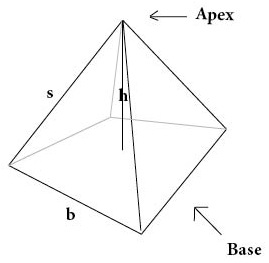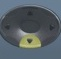## Pyramid

##### Pyramid = Three-Dimensional Shape with Polygonal Base Connecting to a PointBasics

h = height
b = base = distance along a side of pyramid’s base
s = slant height= distance along pyramid’s side
Apex = point or peak to which a pyramid connects
Base = foundation of pyramid
p = perimeter of base
B = area of base
SA = Surface Area = area covering surface, including base, of pyramid (measured in square units)
LA = Lateral Area = area covering surface, excluding base, of pyramid (measured in square units)
V = Volume = space occupied by pyramid (measured in cubic units)

The pyramid pictured above, with triangular sides and a square base, is the type of pyramid probably featured most frequently on the GED.  However, pyramids with bases of other polygonal shapes (triangle, pentagon, etc.) are possible.

Formulas (provided by Mathematics Formula Sheet)

SA =$\bf\displaystyle\frac{1}{2}$ps + B

V =$\bf\displaystyle\frac{1}{3}$Bh

Formulas (NOT provided by Mathematics Formula Sheet)

p = 4b (square Base)
B = b² (square Base)

LA =$\bf\displaystyle\frac{1}{2}$ps

h =$\bf\displaystyle\frac{3V}{B}$

s =$\bf\displaystyle\frac{2(SA-B)}{p}$
Parentheses protect order of operations.

Question
An ancient, square-based pyramid has a base of 600 meters, height 800 meters, and slant height 1000 meters.
What is its surface area?

1560000 square meters

p = 4b
p = 4 × 600 = 2400

B = b²
B = 600² = 360000

SA =$\bf\displaystyle\frac{1}{2}$ps + B

SA =$\bf\displaystyle\frac{1}{2}$*2400*1000+360000 = 1560000

Calculator Click What You See Commentblinker clears screen
4 × 600 4*600 p = 4b2400 p
600600² B = b²
enter 360000 B12× 2400 × 1000 + 360000$\bf\displaystyle\frac{1}{2}$*2400*1000+360000 SA =$\bf\displaystyle\frac{1}{2}$ps + B
###### Pyramid

Question
An ancient, square-based pyramid has a base of 600 meters, height 800 meters, and slant height 1000 meters.  What is its volume?

96000000 cubic meters

B = b²
B = 600² = 360000

V =$\bf\displaystyle\frac{1}{3}$Bh

V =$\bf\displaystyle\frac{1}{3}$*360000*800 = 96000000

Calculator Click What You See Commentblinker clears screen
600600² B = b²360000 B13× 360000 × 800$\bf\displaystyle\frac{1}{3}$*360000*800 V =$\bf\displaystyle\frac{1}{3}$Bh96000000 Answer
###### Pyramid

Question
An ancient, square-based pyramid has a base of 600 meters, height 800 meters, and slant height 1000 meters.
What is its lateral area?

1200000 square meters

p = 4b
p = 4 × 600 = 2400

LA =$\bf\displaystyle\frac{1}{2}$ps

LA =$\bf\displaystyle\frac{1}{2}$*2400*1000 = 1200000

Calculator Click What You See Commentblinker clears screen
4 × 600 4*600 p = 4b2400 p12× 2400 × 1000$\bf\displaystyle\frac{1}{2}$*2400*1000 LA =$\bf\displaystyle\frac{1}{2}$ps1200000 Answer
###### Pyramid

Question
An ancient, square-based pyramid has a base of 300 meters and a surface area of 309000 square meters.  What is its slant height?

500 meters

p = 4b
p = 4 × 300 = 1200

B = b²
B = 300² = 90000

s =$\bf\displaystyle\frac{2(SA-B)}{p}$
s =$\bf\displaystyle\frac{2(309000-9000)}{1200}$ = 500

Calculator Click What You See Commentblinker clears screen
4 × 300 4*300 p = 4b1200 p
300300² B = b²90000 B2309000 – 90001200$\bf\displaystyle\frac{2(309000-9000)}{1200}$ s =$\bf\displaystyle\frac{2(SA-B)}{p}$500 Answer
###### Pyramid

Question
An ancient, square-based pyramid has a base of 300 meters and a volume of 1200000 cubic meters.  What is its height?

40 meters

B = b²
B = 300² = 90000

h =$\bf\displaystyle\frac{3V}{B}$

h =$\bf\displaystyle\frac{3*1200000}{90000}$ = 40

Calculator Click What You See Commentblinker clears screen
300300² B = b²90000 B3 × 120000090000$\bf\displaystyle\frac{3*1200000}{90000}$ h =$\bf\displaystyle\frac{3V}{B}$40 Answer
###### Pyramid

Practice – Questions
1.  An ancient, square-based pyramid has a base of 300 meters, height 400 meters, and slant height 500 meters.  What is its surface area?

2.  An ancient, square-based pyramid has a base of 300 meters, height 400 meters, and slant height 500 meters.  What is its volume?

3.  An ancient, square-based pyramid has a base of 300 meters, height 400 meters, and slant height 500 meters.  What is its lateral area?

4.  An ancient, square-based pyramid has a base of 600 meters and a surface area of 1560000 square meters.  What is its slant height?

5.  An ancient, square-based pyramid has a base of 600 meters and a volume of 96000000 cubic meters.  What is its height?